TitleBeginning Algebra
Answer/Discussion to Practice Problems
Tutorial 25: Practice Test on Tutorials 20 - 24

 Problems 1a - 1b:  Name the quadrant or axis in which the point lies.

 1a.   (2, - 4)Answer: (2, -4) lies in Quadrant IV.

 1b.  (0, 4)Answer: (0, 4) lies on the y-axis.

 Problem 2a:  Determine if each ordered pair is a solution of the given equation.

 2a.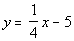;   (0, 5), (4, - 4), (- 4, -6)Answer:

 Let's plug (0, 5) into the equation and see what we get: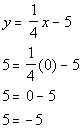This is a FALSE statement, so (0, 5) is a NOT a solution.

 Let's plug (4, -4) into the equation and see what we get: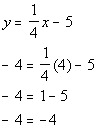This is a TRUE statement, so (4, -4) is a solution.

 Let's plug (-4, -6) into the equation and see what we get: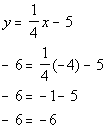This is a TRUE statement, so (-4, -6) is a solution.

 Problem 3a:   Determine whether the equation is linear or not.  Then graph the equation by plotting points.

 3a.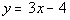Answer:

 If we subtract 3x from both sides, then we can write the given equation as -3x + y = - 4.  Since we can write it in the standard form Ax + By = C, then we have a linear equation.  This means that we will have a line when we go to graph this.

Step 1:  Find three ordered pair solutions.

Solutions:

 x(x, y) -1 y = 3(-1) - 4 = -7 (-1, -7) 0 y = 3(0) - 4 = - 4 (0, - 4) 1 y = 3(1) - 4 = -1 (1, -1)

 Step 2:  Plot the points found in step 1.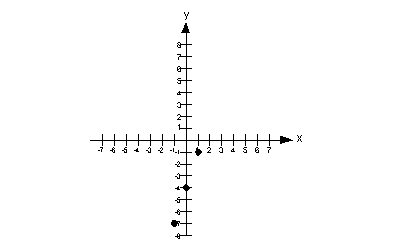Step 3:  Draw the graph.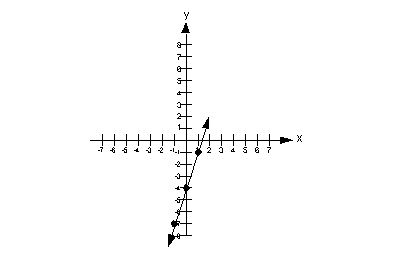Problems 4a - 4b: Graph each linear function using the x- and y- intercepts.

 4a.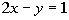Answer:

 Step 1:  Find the x- and y- intercepts. Let's first find the x-intercept.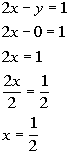*Find x-int. by replacing y with 0   *Inverse of mult. by 2 is div. by 2

 The x-intercept is (1/2, 0).   Next we will find the y-intercept.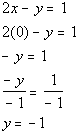*Find y-int. by replacing x with 0   *Inverse of mult. by -1 is div. by -1

 The y-intercept is (0, -1)

 Step 2:  Find at least one more point. We can plug in any x value we want as long as we get the right corresponding y value and the function exists there.  Let's put in an easy number  x = 1: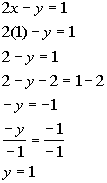*Replace x with 1 *Inverse of add 2 is sub. 2   *Inverse of mult. by -1 is div. by -1

So the ordered pair (1, 1) is another solution to our function.

Note that we could have plugged in any value for x: 5, 10, -25, ...,  but it is best to keep it as simple as possible.

Solutions:

 x y (x, y) 1/2 0 (1/2, 0) 0 -1 (0, -1) 1 1 (1, 1)

 Step 3: Plot the intercepts and point(s) found in steps 1 and 2.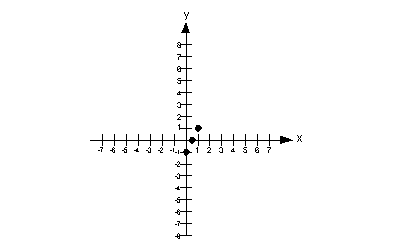Step 4:  Draw the graph.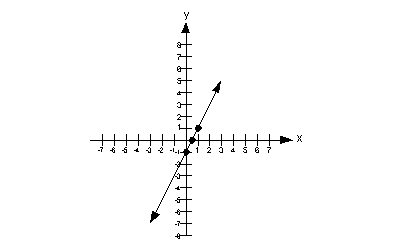4b.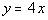Answer:

 Step 1:  Find the x- and y- intercepts. Let's first find the x-intercept.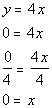*Find x-int. by replacing y with 0 *Inverse of mult. by 4 is div. by 4

 The x-intercept is (0, 0).   Since the x-intercept came out to be (0, 0), then it stands to reason that when we put  in 0 for x to find the y-intercept we will get (0, 0).

 Step 2:  Find at least one more point. Since we really have found only one point, this time we better find two additional solutions so we have a total of three points. We can plug in any x value we want as long as we get the right corresponding y value and the function exists there.  Let's put in an easy number x = 1: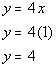*Replace x with 1

 So the ordered pair (1, 4) is another solution to our function.    Let's put in another easy number x = -1: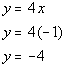*Replace x with -1

So the ordered pair (-1, -4) is another solution to our function.

Note that we could have plugged in any value for x: 5, 10, -25, ...,  but it is best to keep it as simple as possible.

Solutions:

 x y (x, y) 0 0 (0, 0) 1 4 (1, 4) -1 -4 (1, -4)

 Step 3: Plot the intercepts and point(s) found in steps 1 and 2.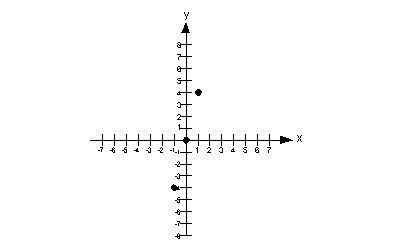Step 4:  Draw the graph.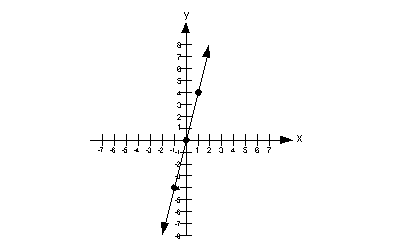Problem 5a: Graph the linear equation.

 5a.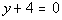Answer:

 If we subtract 4 from both sides, we would get y = - 4, which would fit the form y = c. With that in mind, what kind of line are we going to end up with? Horizontal.

Step 1:  Find the x- and y- intercepts
AND
Step 2:  Find at least one more point

Since this is a special type of line, I thought I would talk about steps 1 and 2 together.

It doesn't matter what x is, y is always - 4.  So for our solutions we just need three ordered pairs such that y = - 4.

Note that the y-intercept (where x = 0) is at (0, - 4).

Do we have an x-intercept? The answer is no.  Since y has to be - 4, then it can never equal 0, which is the criteria of an x-intercept.  Also, think about it, if we have a horizontal line that crosses the y-axis at - 4, it will never ever cross the x-axis.

So some points that we can use are (0, - 4), (1, - 4) and (-1, - 4).  These are all ordered pairs that fit the criteria of y having to be 4.

Of course, we could have used other solutions, there are an infinite number of them.

Solutions:

 x y (x, y) 0 - 4 (0, - 4) 1 - 4 (1, - 4) -1 - 4 (-1, - 4)

 Step 3:  Plot the intercepts and point(s) found in steps 1 and 2.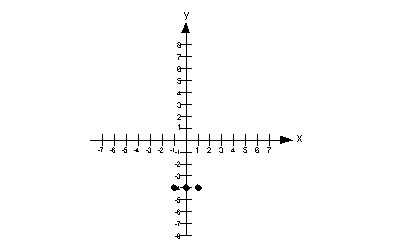Step 4:  Draw the graph.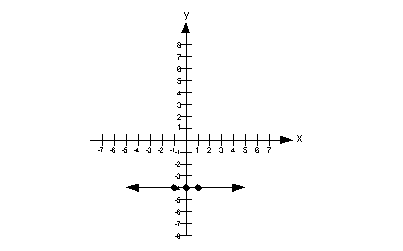Problem 6a - 6b: Find the slope of each line if it exists.

 6a.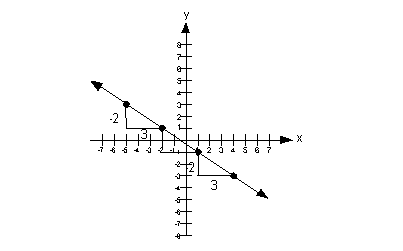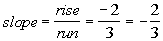6b.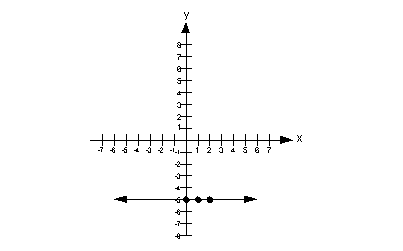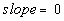Problems 7a - 7b: Find the slope of the straight line that passes through the given points.

 7a.  (-7, 3) and (4, -1)Answer: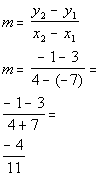*Plug in x and y values into slope formula *Simplify

 The slope of the line is -4/11.

 7b.  (5, 0) and (5, 4)Answer: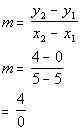*Plug in x and y values into slope formula *Simplify

 The slope of the line is undefined.

 Problem 8a:  Graph the inequality.

 8a.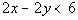Answer:

 Step 1:  Graph the boundary line.

 I'm going to use the intercepts to help me graph the boundary line.  Again, you can use any method that you want, unless the directions say otherwise. When I'm working with only the boundary line, I will put an equal sign between the two sides to emphasize that we are working on the boundary line.  That doesn't mean that I changed the problem. When we put it all together in the end, I will put the inequality back in. x-intercept: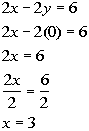*Replace y with 0   *Inverse of mult. by 2 is div. by 2 *x-intercept

 x-intercept is (3, 0)   y-intercept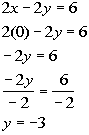*Replace x with 0   *Inverse of mult. by -2 is div. by -2 *y-intercept

 y-intercept is (0, -3).   Plug in 1 for x to get a third solution: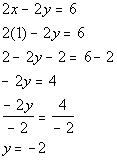*Replace x with 1 *Inverse of add 2 is sub. 2   *Inverse of mult. by -2 is div. by -2

(1, -2) is another solution on the boundary line.

Solutions:

 x y (x, y) 3 0 (3, 0) 0 -3 (0, -3) 1 -2 (1, -2)

Since the original problem has a <, this means it DOES NOT include the boundary line.

So are we going to draw a solid or a dashed line for this problem?
It looks like it will have to be a dashed line.

Putting it all together, we get the following boundary line for this problem: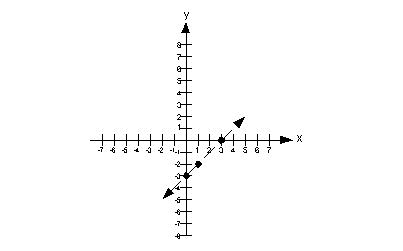Step 2:  Plug in a test point that is not on the boundary line.

 Note how the boundary line separates it into two parts.  An easy test point would be (0, 0).  Note that it is a point that is not on the boundary line. In fact, it is located above the boundary line.  Let's put (0, 0) into the original problem and see what happens: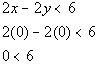*Replacing x and y with 0 *True statement

 Step 3:  Shade in the answer to the inequality.

 Since our test point (0, 0) made our inequality TRUE, this means it is a solution.  Our solution would lie above the boundary line.  This means we will shade in the part that is above it. Note that the gray lines indicate where you would shade your final answer.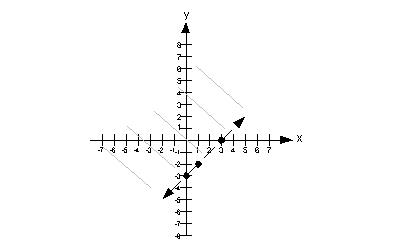Last revised on July 31, 2011 by Kim Seward.
All contents copyright (C) 2001 - 2011, WTAMU and Kim Seward. All rights reserved.